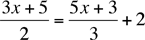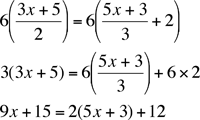SEARCH HOMEMath Central Quandaries & QueriesSubject: math question Name: jaclyn Who are you: Student (3x+5) / 2 = (5x+3) / (3)+ 2Hi Jaclyn,

I assume that you want to solve for x whenI would look at the two fractions and find the least common denominator. In this case it is 6. Now multiply both sides of the equation by this least common denominator.Solve for x.

PennyMath Central is supported by the University of Regina and The Pacific Institute for the Mathematical Sciences.